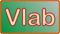onlinechannel15b:   Rating curve in a prismatic channel using the Manning equationDefinition sketch for a prismatic channel
Formulas:

P = b + 2y(1 + z2)1/2
T = b + 2zy
A = y(b + zy)
R = A/P
D = A/T
Q = (C/n) AR2/3S1/2
v = Q/A
F = v/(gD)1/2

INPUT DATA:

Stream or river (optional):

Select:
 SI units (metric) U.S. Customary units

Bottom width b :

Flow depth y :

Side slope z1 :

Side slope z2 :

Manning's n :

Bottom slope S :

INTERMEDIATE CALCS:

Units selected:

Grav. accel. g:

Constant C:

Wetted perimeter P:

Top width T:

Flow area A:

Hydraulic depth D:

OUTPUT:

Stream or river (optional):

Discharge
Q:

Flow velocity
v:

Froude number
F:

Exponent of the rating
β:

Neutrally stable Froude number
Fns:

Vedernikov number
V:

 N y Q A 0 0 0 0

Discharge-area rating:   Q = α A β

Coefficient:  α =

Exponent:  β =

Neutrally stable Froude number:  Fns =

Your request was processed at  09:13:34 pm on January 28th, 2022   [ 220128  21:13:34 ].

Thank you for running onlinechannel15b.php.   Please call again.